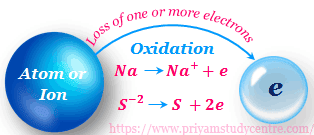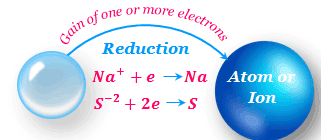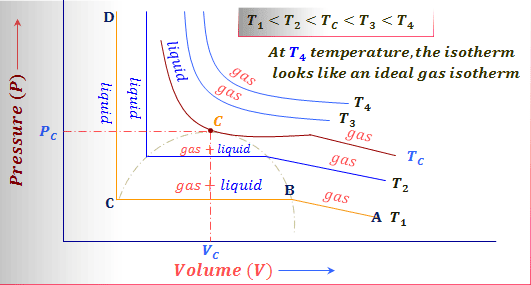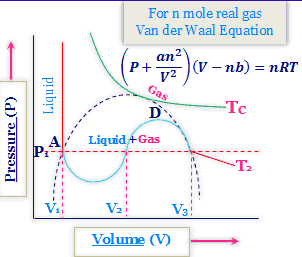March 2019

Oxidation

Oxidation has been classically defined as the combination of oxygen or any other electronegative element with another element or compound or as the removal of hydrogen or any other electropositive element from a chemical compound.Process of oxidation

Combination of oxygen means oxidation

1. When carbon burns in oxygen to produce carbon dioxide. Here oxygen is combined with carbon to produce carbon dioxide and carbon is considered to have been oxidized.
C + O₂ ⇆ CO₂
2. The magnesium metal reacts with chlorine to produce magnesium chloride. In this reaction, magnesium is considered to have been oxidized because magnesium combines with oxygen to produced magnesium oxide.
2Mg + O₂ ⇆ 2MgO
3. When Ferrous Oxide heated in oxygen to produce Ferric Oxide. Here Ferrous oxide is considered to have oxidized.
4FeO + O₂ ⇆ 2Fe₂O₃

Combination of an electronegative element

When Chlorine gas passes through the colorless ferrous chloride solution it forms #0050ff Ferric chloride. Here ferrous chloride oxidized by the addition of electronegative element chlorine.
2FeCl₂ + Cl₂ ⇆ 2FeCl₃

Removal of Hydrogen means oxidation

1. Manganese dioxide reacts with concentrated hydrochloric acid to produce greenish-#0050ff chlorine gas.
4HCl + MnO₂ ⇆ MnCl₂ + Cl₂ + H₂O
Here hydrogen can remove from HCl to form chlorine gas, hence the removal of hydrogen from HCl indicated that HCl can be oxidized.
2. Hydrogen Sulphide reacts with chlorine to produce Sulphur.
H₂S + Cl₂ ⇆ 2HCl + S
In the above reaction, hydrogen can remove from hydrogen sulfide. Thus hydrogen sulfide oxidized to form sulfur.

Removal of electro-positive elements

Potassium iodide reacts with Hydrogen peroxide to produce iodine.
2KI + H₂O₂ ⇆ 2KOH + I₂
In the above reaction, electro-positive metal K can remove from KI to from iodine. Thus KI can be oxidized.

Reduction

The reduction has been classically defined as the combination of hydrogen or any other electropositive element with another element or compound or as the removal of oxygen or any other electronegative element from a chemical compound.Process of reduction

Addition of Hydrogen means the reduction

1. Bromine can react with hydrogen to produce HBr.
H₂ + Br₂ ⇆ 2HBr
In the above reaction, Bromine can combine with hydrogen to produce hydrogen bromide. Thus bromine can be reduced.
2. Hydrogen Sulphide reacts with chlorine to produce Hydrogen chloride and Sulphur.
H₂S + Cl₂ ⇆ HCl + S
In the above reaction, chlorine can combine with hydrogen. Thus chlorine can be reduced.

Addition of electro-positive elements

2K₃[Fe(CN)₆ + 2KOH + H₂O₂ ⇆ 2K₄[Fe(CN)₆] + 2H₂O + O₂
In the above reaction Potassium ferricyanide combination with electro-positive element K to produce potassium ferrocyanide. Thus potassium ferricyanide can be reduced.

Removal of Oxygen means the reduction

When hydrogen can pass through black color heated CuO, Oxygen can remove from CuO to form red color Cu. Thus CuO can be reduced.
CuO + H₂ ⇆ Cu +H₂O

Removal of electro-negative elements

Sulfur dioxide gas passes through a #0050ff ferric sulfate solution it turns greenish ferrous sulfate solution.
Fe₂(SO₄)₃ + 2SO₂ + H₂O ⇆ 2FeSO₄ + 2H₂SO₄
In the above reaction ferric sulfate removes sulfate to form ferrous sulfate. Thus ferric sulfate reduced to form ferrous sulfate.

Oxidation

According to the Electronic concept, oxidation is a process that results in the loss of one or more electrons by atoms or ions. Therefore an oxidizing agent is one that gains electrons and is then reduces to a lower valence.Electronic Concept of Oxidation

Loss of Electron means oxidation

Na, K, Ca, Al, etc. can losses one or more electrons to form their respective cations.
Na → Na⁺ + e

K → K⁺ + e

Ca → Ca⁺² + 2e

Al → Al⁺³ + 3e
All the above reaction the atoms can lose one or more electrons to form respective cations. Thus all the above reaction is oxidation reaction and all these atoms are oxidized.

Loss of Electron by Cation

Fe⁺²(Ferrous), Cu⁺(Cuprous), Sn⁺²(Stannous) ion converted Fe⁺³(Ferric), Cu⁺²(cupric), Sn⁺⁴(Satanic) ion by losses one or more electrons. Thus these cations can be oxidized.
Fe⁺² → Fe⁺³ + e

Cu⁺ → Cu⁺² + e

Sn⁺² → Sn⁺⁴ + e

Loss of Electron by Anion

Cl⁻(Chloride), Br⁻(Bromide), I⁻(Iodide), S⁻²(Sulphide) anion can losses one or more electrons to form respective elements. In this reaction, these anions can be oxidized.
2Cl⁻ → Cl₂ + e 2Br⁻ → Br₂ + e

2I⁻ → I₂ + e

S⁻² → S + 2e
From the above Examples, we understand that oxidation is,
1. Oxidation is a process for the conversion of the metal atom to cation.
2. Oxidation is a process for conversation lower valence cation to higher valence cation.
3. Oxidation is a process for the conversation of anion to neutral atom.

Reduction

According to Electronic concept, the reduction is a process that results in the gain of one or more electrons by atoms or ions. Therefore reducing agent is one that loses electrons and is oxidized to a higher valence.Electronic Concept of Reduction

Gain of Electron means the reduction

Na⁺, Cu⁺², Fe⁺², Fe⁺³ cations can gain one or more electrons to form respective metals.
Na⁺ + e → Na

Cu⁺² + 2e → Cu

Ca⁺² + 2e → Ca

Al⁺³ + 3e → Al

Lower Valence cation from Higher Valence Cation

Fe⁺³(Ferric), Cu⁺²(cupric), Sn⁺⁴(Satanic) ion gaining one or more electrons to form Fe⁺²(Ferrous), Cu⁺(Cuprous), Sn⁺²(Stannous) ion. Thus these cations can be reduced.
Fe⁺³ + e → Fe⁺²

Cu⁺² + e → Cu⁺

Sn⁺⁴ + e → Sn⁺²

Accept of electrons by an atom

Chlorine, Bromine, sulfur Oxygen can accept an electron to form a respective anion. In these reactions, these anions can be reduced.
Cl₂ + 2e → Cl⁻

S + 2e → S⁻²

O₂ +4e → O⁻²
From the above discussion, it is clear that the reduction reaction is the reverse reaction of oxidation.

Ideal gas law problems solutions

The gases are characterized by the lack of definite volume or shape. In the gaseous state, the matter has the properties of filling completely any available space to a uniform density.
Low density and high compressibility are also pronounced characteristics of gases.Ideal gas law
Problem
Explain an ideal gas cannot be liquefied?
Solution
For gas to liquefy, it is necessary for the molecules to come close to each other. For that, the gas should have the intermolecular attraction.
But in an ideal gas, it is assumed that there is no intermolecular attraction and hence it can not be liquefied.
Further putting the condition of the critical state of the ideal gas equation, PV = RT, it is possible to show that the critical temperature(Tc) for an ideal gas is 0⁰ K.
For ideal gas law,
PV = RT
or, (dP/dV)T = - RT/V²

At the critical state (dP/dV)T = 0

hence (- RTc/Vc²) = 0
or, Tc = 0⁰ K
Thus to liquefy an ideal gas, it is necessary to bring the temperature below 0⁰ K and it is not possible and so the ideal gas cannot be liquefied.
Problem
Find the density of ammonia gas at 100⁰ C, when confined by the pressure of 1600 mm Hg.
Solution
The ideal gas equation is,
PV = nRT
or, PV = (g/M)RT
or, PV = (g/V)(RT/M)
or, P = dRT/M
or, d = PM/RT
In the above problem molecular weight M = 17 gm mol⁻¹,
Pressure P = 1600 mm Hg = 1600/760 atm,
R = 82.1 cc atm mol⁻¹ K⁻¹ and T = (273 +100) K

Thus density (d) = (17 × 1600)/(760 × 82.1 × 373)
= 0.00117 gm/cc
Problem
Express the coefficient of thermal expansion(α) of a gas. Show that α depends on the temperature for an ideal gas.
Solution
Coefficient of thermal expansion(α) is defined as, α = (1/V)[dV/dT]P

Ideal gas equation for 1 mole gas is,PV = RT
Hence [dV/dT]P = R/P
Thus α = (1/V) × (R/P) = (R/PV) = 1/T
This means all the gases have the same coefficient of thermal expansion.
Problem
The compressibility factor Z of a gas is given by Z = PV/RT. What is the importance of this expression?
Solution
The expression of Z provides the measures of non-ideality of a gas. It is the single parameter by the extent of the non-ideality of gas that could be measured.
Thus when Z = 1, the gas behaves ideally.
But when Z ≠ 1, the gas behaves non-ideal and the departure of the value Z from one measures the extent of non-ideality of the gas.
When Z ㄑ1, PV ㄑRT
That is, PVㄑPi Vi
Thus the gas becomes more compressible than ideal gas.

Again when Z 〉1,
the gas is less compressible than the ideal gas.

Problem
Show that Z is always greater than 1 for a gas obeying the equation P(V - b) = RT. What does the result signify?
Solution
The equation is given as,
P(V - b) = RT
or, PV = RT + bP
or, (PV/RT) = 1 + (Pb/RT)
or, Z = 1 + (Pb/RT)

From the above relation, Pb/RT is (+)ve thus Z 〉1.
Problem
Why an ideal gas cannot have a reduced equation of state?
Solution
The reduced equation of state is expressed in terms of reduced variables like reduced temperature (θ) reduced pressure (π) and reduced volume (φ).
These variables are defined as, θ = T/Tc
π = P/Pc
φ = V/Vc
where Tc, Pc, and Vc are the critical temperature, pressure, and volume respectively.

The ideal gas is represented by the equation,
PV = RT for 1 mole.
But the critical temperature of the ideal gas is 0° K, hence the critical state is not attained by the ideal gas since 0°K is unattainable.
Since critical Constants (Pc, Tc, and Vc) are not available for an ideal gas, so the reduced variables (π, θ, φ) are also not available for an ideal gas.
Thus reduced equation of state cannot be constructed for an ideal gas.
Problem
How the root means square velocity for oxygen compares with that of the hydrogen?
Solution
The root means square velocity of hydrogen is four-times that of oxygen.
Problem
Calculate the pressure excreted on the walls of a 3 liter of the flask when 7 gms of nitrogen are introduced into the same at 27°C.
Solution
We have, PV = nRT
or, PV = (g/M)RT (molecular wt. of N₂ = 28)
∴ P = (7/28) × (0.082 × 300)/3
= 2.05 atm

Here R = 0.082 lit atm mol⁻¹ K⁻¹
Problem
What is the molecular weight of a gas, 12.8 gms of which occupy 10 liters at a pressure of 750 mm and at 27° C?
Solution
31.91 gm mol⁻¹
Problem
Calculate the total kinetic energy of 0.5 mol of an ideal gas at 273 K.
Solution
Total kinetic energy, = n (3RT/2)
= 0.05 {(3/2) × 8.314 × 273}

= 1702 J

Chemical equilibrium problems solutionsChemical equilibrium
Problem
H₂ (g) + ½ S₂ (g) = H₂S (g), KP₁
H₂ (g) + S₂ (g) = 2H₂S (g), KP₂
Show that, KP₂ = (KP₁)²
Solution
For the first reaction,
ΔG⁰₁ = - RT ln KP₁

For the second reaction,
ΔG⁰₂ = - RT ln KP₂
Since, ΔG⁰₂ = 2ΔG⁰₁
Therefore, it follows that - RT ln KP₂ = - 2RT ln KP₁

∴ Kp₂ = (Kp₁)²
Problem
Use Gibbs - Helmholtz equation to derive the Vant Hoff reaction isochore. What condition do you expect a linear relationship between log k and 1/T?
Solution
The Gibbs - Helmholtz equation is,
ΔG⁰ = ΔH⁰ + T[d(ΔG⁰)/dT]P
Zero superscript is indicating the stranded values.
or, - (ΔH⁰/T² ) = -(ΔG⁰/T² ) + 1/T[d(ΔG⁰)/dT]p
or, - (ΔH⁰/T² ) = [d/dT(ΔG⁰/T)]p
Again Van't Hoff isotherm is,
- RT lnKp = ΔG⁰
or, - R lnKp = ΔG⁰/T
Differentiating with respect to T at constant P,
- R [dlnKP/dT]p = [d/dT(ΔG⁰/T)]p
Comparing the above two equation we have,
 dlnKp/dT = (ΔH⁰/T²
Greater the value of ΔH⁰, the faster the equilibrium constant(Kp)changes with temperature(T), ΔH⁰ should remain constant for the linear plot of log Kp vs 1/T.
Problem
How does the equilibrium constant for a reaction, 2A + 3B ⇆ 4C + Heat, change when (i) pressure is increasing (ii) temperature is decreasing (iii) a catalyst added?
Solution

1. Equilibrium constant remains the same when P is increased. The reaction is exothermic, hence ΔH = (-) ve so the equilibrium constant is increased with decreases of temperature.
2. Equilibrium constant remains the same though a catalyst is added.
ΔG⁰ = - RT lnKa
but ΔG⁰ is not changed due to the addition of catalyst as the latter does not participate in the reaction.
3. The initial state and the final state of a chemical reaction remains the same whether the catalyst is added or not. Hence the (Ka) remains unchanged.

Problem
For a reaction,2A + B ⇆ 2C, ΔG⁰(500 K) = 2 KJ mol-1. Find the Kp at 500 K for the reaction A + ½B ⇆ C.
Solution
ΔG⁰ (500 K) for the reaction,
A + ½B ⇆ C
= (2 KJ mol-1)/2
= 1 KJ mol-1
The relation is, ΔG⁰ = - RT lnKp
or, 1 = - (8.31 × 10-3) × (500) lnKp
or, lnKp = 1/(8.31 × 0.5)
= 0.2406
∴ Kp = 1.27
Problem
Justify or criticize the following: "The equilibrium yield of products can not change if the equilibrium constant is kept fixed."
Solution
This statement is not correct.
Equilibrium yield of the product is changed if pressure is changed (Δγ ≠ 0), if an inert gas is added at constant P (Δγ ≠ 0), and any of the reacting components are added a depleted.
For example, N₂ + 3 H₂ ⇆ 2NH₃
The equilibrium yield of NH₃ is increased if P is increased though equilibrium constant kept fixed.
Problem
Justify or criticize the following: the heat of reaction is the same whether a catalyst is used or not.
Solution
H is a state function hence ΔH (heat of a reaction) does not change if the initial state and final state of a process are the same. A catalyst cannot change the initial and final state of a chemical reaction, hence ΔH remains the same whether a catalyst is used or not.
Therefore the statement is correct.

Definitions of critical constants of a gas

A gas can be liquefied by lowering temperature and increasing pressure. But the influence of temperature is more important.
Most of the real gases are liquefied at ordinary pressure by the suitable lowering of the temperature. But gas cannot be liquefied unless its temperature is below a certain value depending upon the nature of the gas whatever high the pressure may be applied.
This temperature of the gas is called its Critical temperature (Tc). Gas can only be liquefied when the temperature is kept below Tc of the gas.

Critical Temperature of a gas

Critical temperature (Tc) is the maximum temperature at which a gas can be liquefied, which is the temperature above which a liquid cannot exist.

Critical Pressure of a gas

Critical pressure (Pc) is the maximum pressure required to cause liquefaction at the temperature (Tc).

Critical Volume of a gas

Critical volume (Vc) is the volume occupied by one mole of a gas at critical temperate (Tc) and critical pressure (Pc).

Andrews isotherms for real gases

In 1869, Thomas Andrews carried out an experiment in which P - V relations of carbon dioxide gas were measured at different temperatures.Critical constant of gas
Following are observed from this graph
1. At high temperatures, such as T4, the isotherms look like those of an Ideal gas.
2. At low temperatures, the curves have altogether different appearances. Consider, for example, a typical curve. As the pressures increase, the volume of the gas decreases (curve A to B).
3. At the point, B liquefaction commences and the volume decreases rapidly as the gas converted to a liquid with much higher density. This conversion takes place at constant pressure P.
4. At point C, liquefaction is complete and thus the CD is evidence of the fact that the liquid cannot be easily compressed. Thus, we note that AB represents the gaseous state, BC, liquid, and vapor in equilibrium, and CD shows the liquid state only.
5. At still higher temperatures we get a similar type of curve as discussed in the above point, except that the horizontal portion is reduced. The Pressure corresponding to this portion is higher than at lower temperatures.
6. At temperatures, Tc the horizontal portion is reduced to a mere point. At temperatures higher then Tc there is no indication of qualification at all.
Thus for every gas, there is a limit of temperature above which it cannot be liquefied, no matter what the pressure is.

Continuity of state for real gases

It appears from the Amagat curve at T there is discontinuity or break during the transformation of gas to liquid. But it is not so. The continuity of the states from the gas to liquid can be explained from the following isotherm ABCD at T₁.
The gas at A is heated to B at constant volume (V) along AB. Then the gas is gradually cooled at constant P along BC, the volume is reduced considerably. The gas is again cooled at constant V until the point D is reached. Nowhere in the process liquid would appear.
At D, the system is a highly compressed gas. But from the curve, this point is the representation of the liquid state. Hence there is hardly a distinction between the liquid state and the gaseous state. There is no line of separation between the two phases. This is known as the principle of continuity of the state.

Critical phenomena and Van der Walls equation

One mole gas Van der Waals equation,
(P + a/V2)(V - b) = RT
or, V3 - (b + RT/P)V2 + (a/P)V - ab/P = 0
This equation has three roots in V for given values of a, b, P and T. It found that either all the three roots are real or one is real and the other two are imaginary.Critical phenomena

Characteristics of the above isotherm

1. At higher temperatures and higher volume regions, the isotherms look much like the isotherms for an Ideal gas.
2. At the temperature lower than Tc the isotherm exhibits a maximum and a minimum. For certain values of pressure, the equation gives three roots of volume as V1, V2, and V3 at pressure P1.
The section AB and ED of the Van der Waals curve at T1 can be realized experimentally. ED represents supersaturated or supercooled vapor and AB represents superheated liquid. Both of these states are meta-stable.
These states are unstable in the seance that slight disturbances are sufficient to cause the system to revert spontaneously into the stable state with the two phases present in equilibrium.
3. The section BCD of the Van der Waals isotherms cannot be realized experimentally. In this region the slope of the P - V curve is positive. Increasing or decreasing the volume of such a system would increase or decrease in pressure. The line BCD represents the metastable state.

Critical constants in terms of Van der Waals constants

Again with the increase of temperature the minimum and maximum points come close to each other and at the critical point (C) both maximum and minimum coalesce. The slope and curvature both become zero at this point.
Thus the mathematical condition of critical point is,
(dP/dV)T = 0 and (d2P/dV2)T = 0
Van der Waals equation for 1 mole gas is,
(P + a/V2)(V - b) = RT
or, P = RT/(V - b) - a/V2

Differentiating Van der Waals equation with respect to V at constant T,
We get Slope,
(dP/dV)T = - {RT/(V - b)2} + 2a/V3
And Curvature,
(d2P/dV2)T = {2RT/(V - b)3} - 6a/V4
Hence at the critical point,
- {RTc/(Vc - b)2} + 2a/Vc3 = 0
or, RTc/(Vc - b)2 = 2a/Vc3
and {2RTc/(Vc - b)3} - 6a/Vc4 = 0
or, 2RTc/(Vc -b)3 = 6a/Vc4
Thus, (Vc - b)/2 = Vc/3

∴ Vc = 3b

Putting the value of Vc = 3b in RTc/(Vc - b)2 = 2a/Vc3.
We have, RTc/4b2 = 2a/27b3

∴ Tc= 8a/27Rb

Again the Van der Walls equation at the critical state,
Pc = RTc/(Vc - b) - a/Vc2
Putting the value of Vc and Tc,

∴ Pc = a/27b2

Problem
The Critical constants for water are 647 K, 22.09 MPa and 0.0566 dm3mol-1. Calculate the value of Van der Waals constants a and b.
We have Tc = 647 K, Pc = 22.09 Mpa = 22.09 × 103 kPa and Vc = 0.0566 dm3 mol-1
Thus, b = Vc/3 = (0.0566 dm3 mol-1)/3
∴ b = 0.0189 dm3 mol-1
a = 3 Pc Vc2
= 3 (22.09 × 103 kPa)(0.0566 dm3 mol-1)2
∴ a = 213.3 kPa mol-2

Compressibility factor at the critical state

The Critical coefficient is defined as,
RTc/PcVc
Thus the value of Critical Coefficient,
= {R × (8a/27Rb)}/{(a/27b2) × 3b}
= 8/3
= 2.66
Thus the value of compressibility factor at the critical state (ZC) = PCVC/RTC
= 3/8
= 0.375

Van der Waals constants in terms of critical constants

Van der Waals constants can be determined from critical constants Tc and Pc of the gas. Vc in the expression is avoided due to difficulty in its determination.
We have, b = Vc/3 but, PcVc/RTCc = 3/8
or, Vc = (3/8) × (RTc/Pc)
∴ b = (1/8)(RTc/Pc)
Again, a = Pc × 27b2 = 3 × Pc × (3b)2
= 3 PcVc2
= 3Pc × (3RTc/8Pc)2

∴ a = (27/64)(R2Tc2/Pc)
Problem
Calculate Van der Waals constants for ethylene. (TC = 280.8 K and PC = 50 atm).
a = 0.057 lit mol-1 and b = 4.47 lit2 atm mol-2
Problem
Argon has (TC = - 122°C, PC = 48 atm). What is the radius of the argon atom?